`All Packages  Class Hierarchy  This Package  Previous  Next  Index`

Class w3c.xmlOnline.tree.Node

```java.lang.Object
|
+----w3c.xmlOnline.tree.Node
```

public class Node
extends Object
Base type for all nodes in an XML tree.

Version:
\$Id: w3c.xmlOnline.tree.Node.html,v 1.7 1997/06/09 22:26:00 bbos Exp \$
Author:
Bert Bosparent
The parent in the XML tree, or nullseqnoNode(Node, int)
Create a new Node with the given parent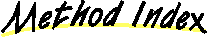addID(String, Element)
Add the caller to the idTable of the root of this tree, with the given ID as key.dumpTree(PrintStream)
A method to write out the content of the node in correct XML synyax.findID(String)
Return the element with a certain ID in the tree around this element.findXPTR(String, int)
Return element pointed to by a TEI xptr.getNodeByNumber(int)
Get the node with seqno n from the subtree below this node.ids()
Return an enumeration of all IDs in the tree.lastSeqno()
Return the highest seqno in the subtree below this nodenrNodes()
Return the number of nodes in the subtree below this nodeparent()
Return the parent of this node.protect(String)
Encode a string with certain characters replaced by numerical character entities.renumber(int[])
Renumber the nodes.replaceBy(Node)
Replace the current node by the given node.replaceBy(Node, Node)
Replace a child node by a different node.replaceBy(Node, Node[])
Replace a child node by a list of different nodes.replaceBy(Node[])
Replace the current node by the given array of nodes.root()
Return the root of the tree.seqno()
Return the sequence number of this node in the tree.setParent(Node)
Set the parent of this node.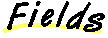parent
``` protected Node parent
```
The parent in the XML tree, or nullseqno
``` protected int seqno
```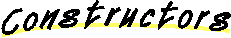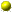Node
``` public Node(Node parent,
int nr)
```
Create a new Node with the given parent

Parameters:
parent - the parent of the node
nr - the sequence number of the node in the tree
Returns:
the new Node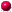setParent
``` public Node setParent(Node node)
```
Set the parent of this node.

Parameters:
node - the node to use as parent
Returns:
selfparent
``` public Node parent()
```
Return the parent of this node.

Returns:
the parent node, or nullreplaceBy
``` public Node replaceBy(Node nodes[])
```
Replace the current node by the given array of nodes. This is done by asking the parent to insert the array in its list of children, and then setting the parent of this node to null, so that it isn't a part of the tree anymore.

Parameters:
nodes - an array of nodes to replace this node in the treereplaceBy
``` protected Node replaceBy(Node child,
Node nodes[])
```
Replace a child node by a list of different nodes.

Parameters:
child - the child node to replace
nodes - the nodes that are to replace the childreplaceBy
``` public Node replaceBy(Node node)
```
Replace the current node by the given node. This is done by asking the parent to insert the node in its list of children, and then setting the parent of this node to null, so that it isn't a part of the tree anymore.

Parameters:
nodes - an array of nodes to replace this node in the treereplaceBy
``` protected Node replaceBy(Node child,
Node node)
```
Replace a child node by a different node.

Parameters:
child - the child node to replace
node - the node that is to replace the childseqno
``` public int seqno()
```
Return the sequence number of this node in the tree.

Returns:
the sequence number of this node in the tree.nrNodes
``` public int nrNodes()
```
Return the number of nodes in the subtree below this node

Returns:
the number of nodes in the subtree below this nodelastSeqno
``` public int lastSeqno()
```
Return the highest seqno in the subtree below this noderenumber
``` protected void renumber(int n_in_out[])
```
Renumber the nodes.

Parameters:
n - the starting numberroot
``` public Node root()
```
Return the root of the tree. Recursively ask for the parent until there is none anymore.

Returns:
the root of the tree of this nodegetNodeByNumber
``` public Node getNodeByNumber(int n)
```
Get the node with seqno n from the subtree below this node.

Parameters:
n - the seqno to look for
Returns:
the node with seqno n in the subtree, or nulladdID
``` protected Node addID(String ID,
Element elt)
```
Add the caller to the idTable of the root of this tree, with the given ID as key. An element that has an attribute called "ID" will use this method to add itself to the idTable of its root element. This call percolates up the tree until it reaches a Root element.

Parameters:
ID - the ID to use as key (value of ID attribute)
elt - the element to add
Returns:
thisfindID
``` public Node findID(String ID)
```
Return the element with a certain ID in the tree around this element. The request is passed up the tree to the Root, which will look up the ID in its hash table.

Parameters:
ID - the ID to look for
Returns:
the element with that ID, or nullids
``` public Enumeration ids()
```
Return an enumeration of all IDs in the tree. If this Root is not the root of the tree, it will pass the request up to the real root.

Returns:
an enumeration of all IDs in the treefindXPTR
``` public Node findXPTR(String xptr,
int n)
```
Return element pointed to by a TEI xptr. The passed string is interpreted as a TEI xptr and the instructions in it are followed to find the element it points to. Only the first part is interpreted, the rest of the string is passed on recursively to the element addressed by the first part. The extra argument indicates which of the addressed elements (in case there is more than one) should be returned.

Parameters:
xptr - the TEI xptr
n - which of the set of addresses element is returned (n>=0)
Returns:dumpTree
``` public void dumpTree(PrintStream out)
```
A method to write out the content of the node in correct XML synyax. Subclasses must overide this method.

Parameters:
out - the PrintStream to write onprotect
``` public String protect(String s)
```
Encode a string with certain characters replaced by numerical character entities.

Parameters:
s - string to encode
Returns:
the encoded string

`All Packages  Class Hierarchy  This Package  Previous  Next  Index`